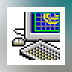# Math solver### Universal Math Solver

Universal Math Solver will solve and explain your algebra or calculus problem step-by-step.### Mathbrain

An authentic MDI software application which is to make graphics solving ordinary differential equations and functions by the analysis of their numerical values.

## Programs for query  ″math solver″### Microsoft Mathematics

Microsoft Mathematics provides a set of mathematical tools that help students get school work done quickly and easily.

... learn to solve equations step ... calculator. Additional math tools help ... , and solve systems of ...... units aware math notation ... to simultaneously solve and ... live, standard math notation, ...### Microsoft Math Add-in for Word

The Microsoft Math Add-in for Microsoft Office Word 2007 makes it easy to create graphs ...

The Microsoft Math Add-in ... calculations, and solve for variables ...### TK Solver Player

5 on 1 vote

TK Solver™ Player for Excel is free software for sharing packaged Excel applications and mathematical models created in TK Solver 5.

TK Solver™ Player for ... in TK Solver 5.0 Premium Edition ... is a collaborative math engine that ...### Master Math Word Problems

5 on 1 vote

Solving word problems is an area where elementary students overwhelmingly display difficulties.

Solving word problems ... difficulties. Master Math Word Problems ... learn new math skills, ...### Fortran Mathematics Library by AKCA

5 on 1 vote

This software is a computer program whose purpose is to fortran mathematics library and some solve linear equation in high performance computing.

... and some solve linear equation ...### Personal Algebra Tutor

The Personal Algebra Tutor is a comprehensive algebra problem solver for solving algebra problems from basic math through college algebra and preCalculus.

... solver for solving ... algebra problems from basic math ... problems! - Solves a wide ...### SBMLToolbox

SBMLToolbox is built on top of libSBML and provides a set of basic functions allowing SBML models to be used in MATLAB.

... MATLAB's Symbolic Math Toolbox; and ...### Smart Math Calculator

CalculatorSmart Math Calculator allows you to define variables and solve math equations.

... variables and solve math equations. ... and solve recursive ... Smart Math Calculator is ...